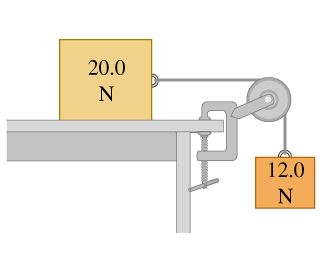# Problem: Two blocks are connected by a very light string passing over a massless and frictionless pulley (Figure ). The 20.0-N block moves 75.0 cm to the right and the 12.0-N block moves 75.0 cm downward.Find the total work done on 20.0-N block if there is no friction between the table and the 20.0-N block.Find the total work done on 20.0-N block if s=0.500 and k=0.325 between the table and the 20.0-N block.Find the total work done on 12.0-N block if there is no friction between the table and the 20.0-N block.Find the total work done on 12.0-N block if s = 0.500 and k = 0.325 between the table and the 20.0-N block.

⚠️Our tutors found the solution shown to be helpful for the problem you're searching for. We don't have the exact solution yet.

###### Problem Details

Two blocks are connected by a very light string passing over a massless and frictionless pulley (Figure). The 20.0-N block moves 75.0 cm to the right and the 12.0-N block moves 75.0 cm downward.

Find the total work done on 20.0-N block if there is no friction between the table and the 20.0-N block.

Find the total work done on 20.0-N block if s=0.500 and k=0.325 between the table and the 20.0-N block.

Find the total work done on 12.0-N block if there is no friction between the table and the 20.0-N block.

Find the total work done on 12.0-N block if s = 0.500 and k = 0.325 between the table and the 20.0-N block.